# Various Simple Image Processing Techniques

Written by Paul Bourke
September 1989

The following describes some basic image manipulation methods. It will assumed that all images are specified as RGB (red, green, blue) components each ranging from 0 to 1. Any other range is simply converted into this range by scaling. The "standard" colour cube is assumed as shown below.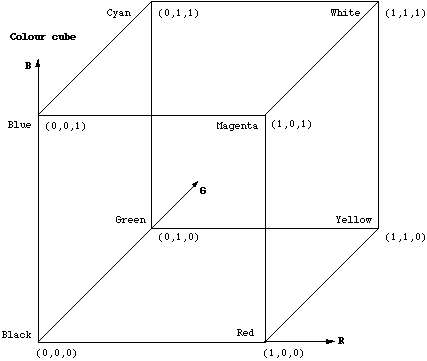Inversion Inverting an image, the RGB value of each pixel is transformed as

R' = 1 - R
G' = 1 - G
B' = 1 - B

While this has a straightforward effect on grey scale images, the result isn't necessarily easy to predict for colour images. Edge detection

There are a whole range of filters that can be applied to images, their basic operation is to take a weighted sum of pixels around the current pixel in order to determine its new value. The simplest edge detector is the filter

```                           -1
-1    4   -1
-1
```

This notation indicates that to compute the new pixel value one takes -1 times the pixel value above, to the right, left and below the current pixel and adds that to 4 times the value of the current pixel. This is repeated for every pixel in the image. It is easy to see that in continuous areas the above results in a total of zero, when the mask spans different colour values it sums to different values.Another filter might be

```                    -1    -1    -1
-1     8    -1
-1    -1    -1
```
or
```                   1/6    4/6   1/6
4/6  -20/6   4/6
1/6    4/6   1/6
```
Colour to grey scale conversion

There are a number of techniques for converting colour images into greyscale, some examples of the common ones are illustrated below by showing how they convert the following image.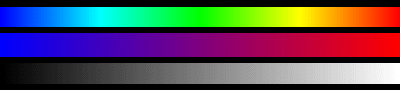The distance of the colour vector in the colour cube is commonly used although it is one of the worst methods. sqrt(red * red + green * green + blue * blue)A simple average of the colours, (red + green + blue) / 3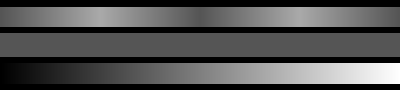A weighted average in common use is ( 3 * red + 4 * blue + 2 * green ) / 9NTSC and PAL uses 0.299 * red + 0.587 * green + 0.114 * blue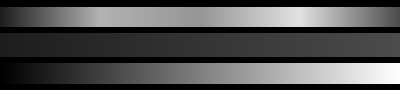ITU-R Recommendation BT.709, "Basic Parameter Values for the Studio and for International Programme Exchange (1990) [formerly CCIR Rec. 709]

grey = 0.2125 * red + 0.7154 * green + 0.0721 * blue

# Bicubic Interpolation for Image Scaling

Written by Paul Bourke
May 2001

There are a number of techniques one might use to enlarge or reduce an image. These generally have a trade off between speed and the degree to which they reduce visual artefacts. The simplest method to enlarge an image by a factor 2 say, is to replicate each pixel 4 times. Of course this will lead to more pronounced jagged edges than existed in the original image. The same applies to reducing an image by an integer divisor of the width by simply keeping every nth pixel. Aliasing of high frequency components in the original will occur. The more general case of changing the size of an image by an arbitrary amount requires interpolation of the colours between pixels.

The simplest method of resizing an image is called "nearest neighbour". Using this method one finds the closest corresponding pixel in the source (original) image (i,j) for each pixel in the destination image (i',j'). If the source image has dimensions w and h (width and height) and the destination image w' and h', then a point in the destination image is given by

i' = i w' / w
j' = j h' / h

where the division above is integer (the remainder is ignored). This form of interpolation suffers from normally unacceptable aliasing effects for both enlarging and reduction of images.

The standard approach is called bicubic interpolation, it estimates the colour at a pixel in the destination image by an average of 16 pixels surrounding the closest corresponding pixel in the source image. Another interpolation technique called bilinear interpolation will not be discussed here, it uses the value of 4 pixels in the source image. There are two methods in common usage for interpolating the 4x4 pixel, cubic B-Spline and a cubic interpolation function, the B-spline approach will be discussed here.

The diagram below introduces the conventions and nomenclature used in the equations. We wish to determine the colour of every point (i',j') in the final (destination) image. There is a linear scaling relationship between the two images, in general a point (i',j') corresponds to an non integer position in the original (source) image. This is position is given by

x = i w' / w
y = j h' / h

The nearest pixel coordinate (i,j) is the integer part of x and y, dx and dy in the diagram is the difference between these, dx = x - i, dy = y - j.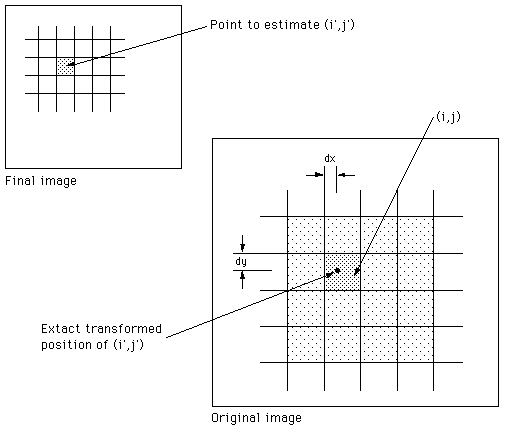The formulae below give the interpolated value, it is applied to each of the red, green, and blue components. The m and n summation span a 4x4 grid around the pixel (i,j).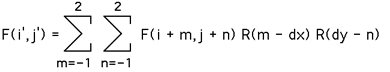The cubic weighting function R(x) is given below.